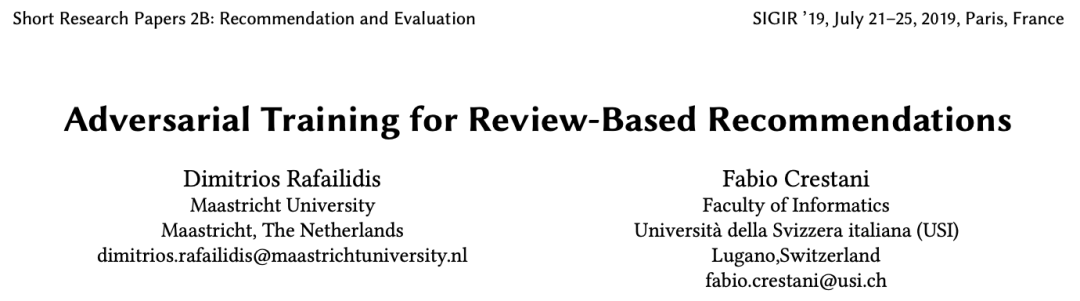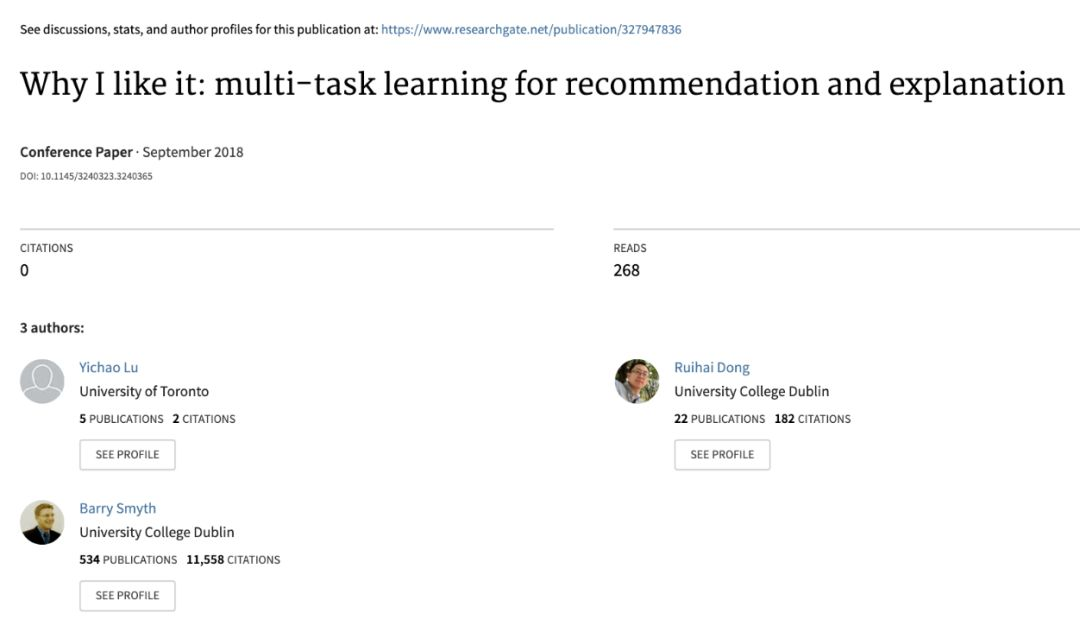## 华人一作论文惨遭SIGIR 2019论文抄袭，网友：我只想知道抄袭者最终下场！

11 月 2 日 新智元### 新智元报道

##### 【新智元导读】Reddit网友爆料：SIGIR 2019的一篇论文涉嫌抄袭，且抄袭的两位作者均为大学教授，而被抄袭的论文其中两位作者是华人学者。SIGIR是信息检索领域最重要的国际顶会，在这样的顶会上发表抄袭的论文，两位教授被认为学术生涯已完。你怎么看，来新智元 AI 朋友圈说说你的观点～

joyyeki表示，最初他以为这只是巧合，因为用两篇论文都采用的对抗性训练也是最近的研究热点，不同小组对同一个问题提出相似的解决方案也正常。然而， 在仔细阅读和比较两篇论文后，他认为SIGIR 2019 的论文抄袭了RecSys 2018的论文。

ACM SIGIR(国际信息检索大会)是信息检索领域最重要的国际学术顶会，在这么权威的会议上发表了一篇抄袭的论文，引发一片质疑声。

• SIGIR 2019论文(抄袭)：Adversarial Training for Review-Based RecommendationsDimitrios Rafailidis是Maastricht大学DKE＆IDS的助理教授。他的主要研究兴趣是机器学习，信息检索，推荐系统和社交媒体挖掘。Dimitrios Rafailidis

https://www.maastrichtuniversity.nl/dimitrios.rafailidis

Fabio Crestani自2007年1月起担任USI信息学学院的正教授。1997年至1999年，他是英国格拉斯哥大学，美国伯克利国际计算机科学研究所和英国卢瑟福·阿普尔顿实验室的博士后研究员。Fabio Crestani

https://search.usi.ch/en/people/4f0dd874bbd63c00938825fae1843200/crestani-fabio

• RecSys 2018论文(被抄袭)：Why I like it: multi-task learning for recommendation and explanationYichao Lu，多伦多大学。Layer 6 AI机器学习科学家。

Ruihai Dong 是都柏林大学（UCD）计算机学院的助理教授。他的研究兴趣广泛在于机器学习和深度学习及其在推荐系统和金融领域的应用。Ruihai Dong

Barry Smyth教授是都柏林大学计算机科学的数字主席，并且是数据分析洞察中心的主任。他从2003年开始担任欧洲人工智能协调委员会（ECCAI）的成员，从2011年开始担任爱尔兰皇家学院的成员。Barry Smyth

https://people.ucd.ie/barry.smyth

SIGIR 2019 论文中提出的模型几乎是对RecSys 2018年论文中模型的复制。理由：

(1)两篇论文都使用了矩阵分解框架上的对抗性序列到序列学习模型。

(2)对于生成器和判别器部分，两篇论文都使用GRU作为生成器，使用CNN作为鉴别器。

(3)优化方法相同，即两部分交替优化。

(4)评估相同，即评估MSE的推荐性能和评估判别器的准确性，以表明生成器已经学会生成相关的评论。

(5)这两篇论文所用的符号和公式看起来极其相似。

(1) SIGIR 2019论文的Section 1： “The Deep Cooperative Neural Network (DeepCoNN) model user-item interactions based on review texts by utilizing a factorization machine model on top of two convolutional neural networks.”

(2) RecSys 2018论文的Section 2： “Deep Cooperative Neural Network (DeepCoNN) model user-item interactions based on review texts by utilizing a factorization machine model on top of two convolutional neural networks.”

(1)SIGIR 2019论文的 Section 2.1：

“The decoder employs a single GRU that iteratively produces reviews word by word. In particular, at time step $t$ the GRU first maps the output representation $z_{ut-1}$ of the previous time step into a $k$-dimensional vector $y_{ut-1}$ and concatenates it with $\bar{U_{u}}$ to generate a new vector $y_{ut}$. Finally, $y_{ut}$ is fed to the GRU to obtain the hidden representation $h_{t}$, and then $h_{t}$ is multiplied by an output projection matrix and passed through a softmax over all the words in the vocabulary of the document to represent the probability of each word. The output word $z_{ut}$ at time step $t$ is sampled from the multinomial distribution given by the softmax."

(2) RecSys 2018论文的Section 3.1.1：

"The user review decoder utilizes a single decoder GRU that iteratively generates reviews word by word. At time step $t$, the decoder GRU first embeds the output word $y_{i, t-1}$ at the previous time step into the corresponding word vector $x_{i, t-1} \in \mathcal{R}^{k}$, and then concatenate it with the user textual feature vector $\widetilde{U_{i}}$. The concatenated vector is provided as input into the decoder GRU to obtain the hidden activation $h_{t}$. Then the hidden activation is multiplied by an output projection matrix and passed through a softmax over all the words in the vocabulary to represent the probability of each word given the current context. The output word $y_{i, t}$ at time step $t$ is sampled from the multinomial distribution given by the softmax.”

(1)SIGIR 2019的Section 2.2：

"Each word of the review $r$ is mapped to the corresponding word vector, which is then concatenated with a user-specific vector. Notice that the user-specific vectors are learned together with the parameters of the discriminator $D_{\theta}$ in the adversarial training of Section 2.3. The concatenated vector representations are then processed by a convolutional layer, followed by a max-pooling layer and a fully-connected projection layer. The final output of the CNN is a sigmoid function which normalizes the probability into the interval of $[0, 1]$", expressing the probability that the candidate review $r$ is written by user $u$.”

(2) RecSys 2018论文的Section 3.1.2：

”To begin with, each word in the review is mapped to the corresponding word vector, which is then concatenated with a user-specific vector that identifies user information. The user-specific vectors are learned together with other parameters during training. The concatenated vector representations are then processed by a convolutional layer, followed by a max-pooling layer and a fully-connected layer. The final output unit is a sigmoid non-linearity, which squashes the probability into the $[0, 1]$ interval."

Reddit网友评论：

thatguydr ：得，这下他们的学术生涯完了，棒！不过有趣的是，怎么能没有自动的方式来查找呢？

entarko ：我一直想知道抄袭者获得怎样的后果了。我review的第一篇论文就是抄袭，我告诉AC但没听说后续有任何事情发生。 据我所知，提交抄袭的论文会被列入黑名单。不一定是永久的，一般也就几年。

slayeriq ：是你吗，Siraj？（之前因涉嫌抄袭论文、拉黑学生被讨伐的网络AI教师）

sid__ ：随着会议规模和论文提交量的扩展，不知道抄袭会不会成为（或者已经）更严重的问题？用机器学习查抄袭可能会更靠谱。

102564 ：他们甚至没有引用第一篇论文（加重了侮辱）。这简直是犯罪...

sparkkid1234 ：我估计他们不敢。真引用就一定会被看出是抄袭啦。

hivesteel ：我没有参加这次会议，但我认为我参加的所有会议和期刊都具有自动检测抄袭的工具。我敢肯定它们效果差强人意，但是从理论上讲确切的匹配绝对应该标记出来。0+0+

### 相关内容1+2+0+0+1+0+0+0+0+0+6+0+0+0+0+0+0+0+1+10+0+0+1+0+0+5+0+0+1+0+
Top